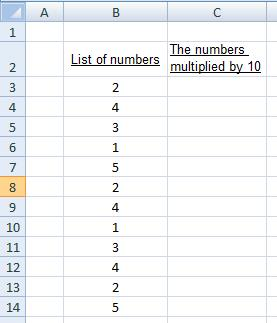Hide

# Using the fill handle to copy formulas

You can download here the worksheet from this video to practice it by yourself!

## When is it useful?

In many occasions there is a need to copy a formula over and over again, with a slight accommodation to it.
Let’s use the example from the video above, and assume you have the following list in your worksheet:If you want to fill column C with the formula that will multiply the list by 10, you have two ways of doing it:

Doing it manually (the hard way):
You can type in cell C3 the formula:
=B3*10
Then type in cell C4 the formula:
=B4*10
Then type in cell C5 the formula:
=B5*10
…and so on until you’ve finished with the list.
(Not practical for long lists.)

Using the fill handle
Type once the formula in cell C3:
=B3*10
Now hit the “Enter” key and select cell C3 again.
Pull downwards the fill handle (the small square at the lower left corner of the active cell), and you have immediately the whole list filled with the correct formulas.

Small tip: instead of pulling the fill handle, try double clicking it!

## How to use the fill handle with formulas?

First prepare your lists, tables, or sets of numbers.
Then type in one instance of the formula you need, and hit Enter. Now go back to that cell, and locate the fill handle, in the direction needed (or double-click it).
It will automatically copy the formula you entered into the new cells and apply the appropriate cell addresses into them from their adjacent cells.

This feature can be used in any direction and basically any kind of formula, allowing you to quickly fill in formulas across your sheets with a minimum of time and effort.

### What next? Important!

As a rule of thumb, try to avoid using numbers inside formulas as we did in the example above, where we have written the number 10 inside the formula.
It's often considered as bad practice, and was used just to help you quickly grasp the way you can copy formulas with the fill handle. Although it seems easy and convenient, try to avoid it.

If you do need to use a constant number inside a formula, then write that number outside, in another cell, and use "absolute reference". Don't worry, this is explained and demonstrated in the next video that teaches absolute and relative referencing.